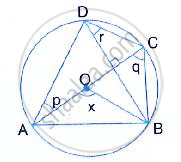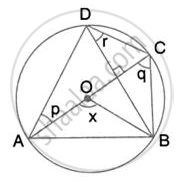Share

# In the Given Figure, Ac is a Diameter of Circle, Centre O. Chord Bd is Perpendicular to Ac. Write Down the Angles P, Q and R in Terms of X . - Mathematics

Course

#### Question

In the given figure, AC is a diameter of circle, centre O. Chord BD is perpendicular to AC. Write down the angles p, q and r in terms of x .#### Solution(Angle at the centre is double the angle at the circumference subtended by the same chord)
⇒  x = 2q and ∠ADB = x/2 ∴= q =x/2

(Angle in a semicircle)

⇒ r + x /2= 90°
⇒ r = 90° - x/2
Again, ∠DAC = ∠DBC

(Angle in the same segment)
⇒ p = 90° - q

⇒ p = 90° - x/2

Is there an error in this question or solution?